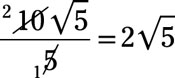##### Pre-Calculus: 1001 Practice Problems For Dummies (+ Free Online Practice)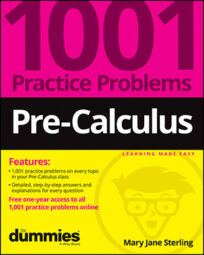The object of solving equations and inequalities is to discover which number or numbers will create a true statement in the given expression. The main techniques you use to find such solutions include factoring, applying the multiplication property of zero, creating sign lines, finding common denominators, and squaring both sides of an equation.

Your challenge is to determine which techniques work in a particular problem and whether you have a correct solution after applying those techniques.

You’ll work with equations and inequalities in the following ways:

• Writing inequality solutions using both inequality notation and interval notation

• Solving linear and quadratic inequalities using a sign line

• Determining the solutions of absolute value equations and inequalities

• Taking on radical equations and checking for extraneous roots

• Rationalizing denominators as a method for finding solutions

Keep in mind that when solving equations and inequalities, your challenges will include

• Factoring trinomials correctly when solving quadratic equations and inequalities

• Choosing the smallest exponent when the choices include fractions and negative numbers

• Assigning the correct signs in intervals on the sign line

• Recognizing the solution x = 0 when a factor of the expression is x

• Determining which solutions are viable and which are extraneous

• Squaring binomials correctly when working with radical equations

• Finding the correct format for a binomial’s conjugate

## Practice problems

1. Describe the graph using inequality notation.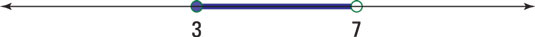Credit: Illustration by Thomson Digital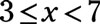The 3 is included in the solution, but the 7 is not.

2. Simplify by rationalizing the denominator.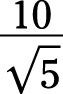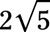Multiply both the numerator and the denominator by the square root of 5: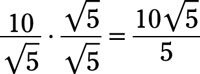Reduce the fraction: